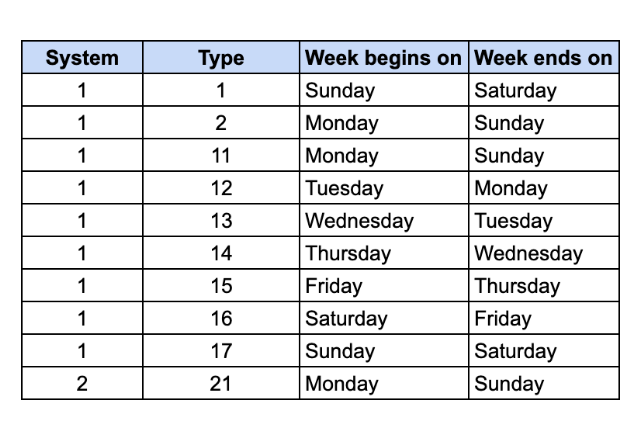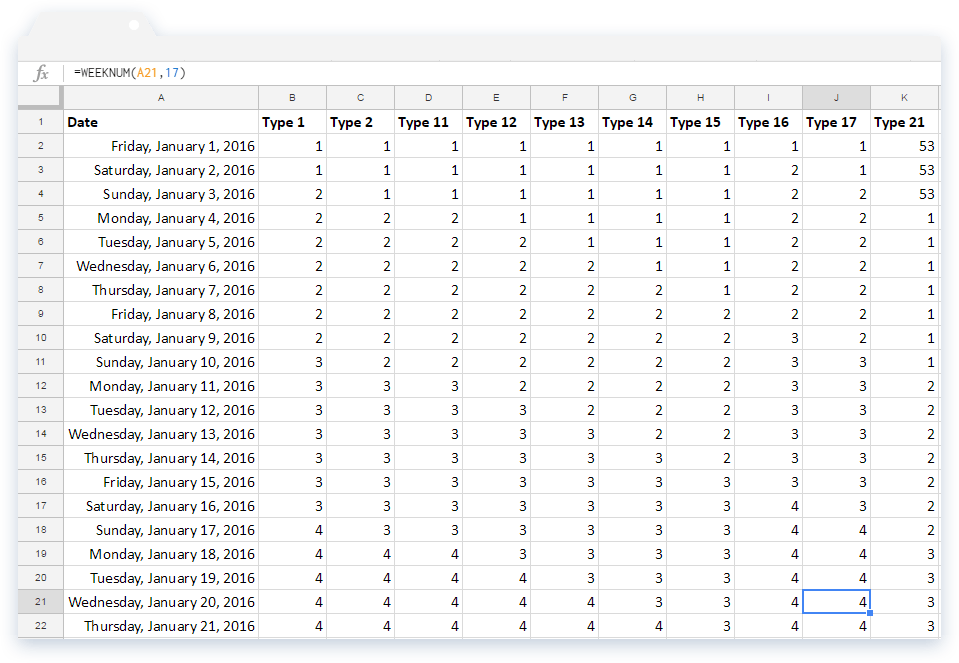# How to use the WEEKNUM formula in Google SheetsIn Google Sheets, if you need to know what specific week of the year a date falls on, you can use the WEEKNUM formula to find out. The week numbers will range from 1 to 53.

### Syntax

WEEKNUM(date, [type])

• date – is the input date that the formula uses to determine the week of the year. This can be a reference to a cell that holds a date, or a formula that returns a date. Not only that, it can even be a number that the Google Sheets can evaluate to a date value.
• type – this is an optional indicator that lets the formula know which day to begin the week. As Google’s documentation explains, the valid inputs for this parameter are 1, 2, 11 through to 17 and 21. There are also two different week numbering systems the formula uses to return the output.
• System 1: The week containing January 1, is week 1. All types except 21 use this system.
• System 2: The week that containing the first Thursday of the year is week 1. Type 21 uses this system, which often goes by the name “European system for numbering weeks”.

Now that we have seen what each system signifies, let’s take a look at what each WEEKNUM formula type stands for. Look at the table below. It shows the week’s start day and end day for each type.### How to use the WEEKNUM Formula

To see the formula in action, take a look at the screenshot below. We have listed a series of dates in column A. The subsequent columns contain the WEEKNUM formulas applied for each available type.Let’s pick type 11 and understand how the formula works. Since this type uses System 1 to calculate week numbers, it considers the week with January 1 as week 1. And, according to the previous table, the week starts on Monday and ends on Sunday for this type. Therefore, week 1 spans from January 1, Friday to January 3, Sunday. Week 2 starts from January 4, as it is Monday, and ends on January 10, Sunday.

Now, let’s look at type 21. This uses System 2, which assumes that the week with the first Thursday of the year is week 1. Taking a quick glance at the data, we know that the first Thursday of the year falls on January 8. And, looking at the table in the first screenshot, the week starts on Monday and ends on Sunday for this type as well. So, week 1 starts on Monday January 4 and ends on Sunday January 10. What about the initial three days that are not part of week 1? The WEEKNUM formula assigns them the last week number of the previous year, which is 53 in this case.

Do you need to check what day of the week it was on a given date? In this case, use the WEEKDAY formula in Google Sheets. Read this blog post to learn how to use it.